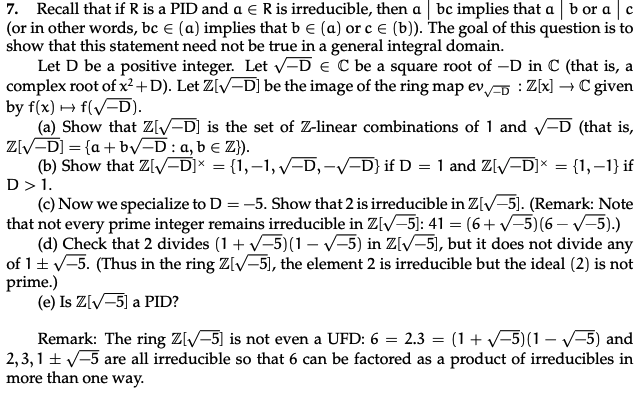Home / Answered Questions / Other / 7-recall-that-if-r-is-a-pid-and-a-r-is-irreducible-then-a-bc-implies-that-a-bor-a-c-or-in-other-word-aw853

# (Solved): 7. Recall That If R Is A PID And A â‚¬ R Is Irreducible, Then A Bc Implies That A Bor A C (or In Oth...7. Recall that if R is a PID and a â‚¬ R is irreducible, then a bc implies that a bor a c (or in other words, bc â‚¬ (a) implies that be (a) or ce (b)). The goal of this question is to show that this statement need not be true in a general integral domain. Let D be a positive integer. Let V-D EC be a square root of -D in C (that is, a complex root of x2 +D). Let Z[VD] be the image of the ring map ev : Z[x] â†’ C given by f(x) + f(V-D). (a) Show that ZIV-D) is the set of Z-linear combinations of 1 and V-D (that is, ZIV-D] = {a+b -D: a,b â‚¬ Z}). (b) Show that Z[V-D]* = {1,-1, V-D-V-D} if D = 1 and Z[V-D]* = {1, -1} if D>1. (c) Now we specialize to D=-5. Show that 2 is irreducible in Z[V-5]. (Remark: Note that not every prime integer remains irreducible in Z[V-5): 41 = (6+-5)(6-7-5).) (d) Check that 2 divides (1 + (-5)(1-V-5) in Z[V-5), but it does not divide any of 15V-5. (Thus in the ring Z[V-5), the element 2 is irreducible but the ideal (2) is not prime.) (e) Is Z[V-5) a PID? Remark: The ring Z[V-5] is not even a UFD: 6 = 2.3 = (1 +/-5)(1-V-5) and 2,3,1 -5 are all irreducible so that 6 can be factored as a product of irreducibles in more than one way.

We have an Answer from Expert